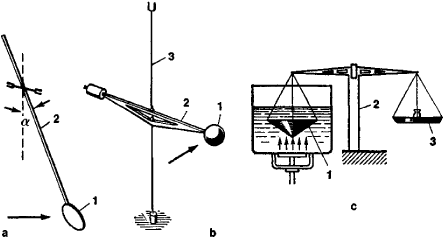A device to measure the acoustic power or intensity of a sound beam by means of the force or torque that the beam exerts on an inserted object or interface. The underlying theory involves the concept of radiation pressure. Such pressure occurs, for example, when a plane sound wave is partially reflected at an interface between two materials, with the nonlinear interaction between the incident and reflected waves giving rise to a steady pressure on the interface. If a narrow beam is incident on the interface and the transmitted wave is fully absorbed by the second material, the magnitude of the radiation force F (area integral of radiation pressure) equals a constant times W/c, where W is the power of the sound beam and c is the sound speed.

A modern acoustic radiometer, used to measure the total power of an ultrasonic sound beam in water and other liquids, employs a vane suspended in the fluid in such a manner that its displacement in a direction normal to its face is proportional to the net force pushing on its front face. The vane is ideally of dimensions somewhat larger than the incident beam's diameter, so that the encountered force is associated with the entire incident beam. To eliminate the possibility of sound being reflected toward the transmitting transducer, the vane is oriented at 45° to the incident sound beam. The vane's horizontal displacement is made to be proportional to the imposed force by fastening the vane at one end of a long pendulum whose rotation from the vertical is opposed by the effect of gravity, such that the apparent spring constant for displacement in a direction at 45° to the face is approximately Mg/L, where M is the apparent mass of the vane (corrected for the presence of water), g is the acceleration of gravity, and L is the length of the pendulum. A nonlinear acoustics theory for such a circumstance yields a proportionality relation between the net horizontal radiation force on the vane and the acoustic power associated with the incident beam. Because the deflection of the vane is proportional to the radiation force, the acoustic power can be determined. See Buoyancy, Pendulum

The concept of the vane device evolved from that of the Rayleigh disk, which was a circular disk that could rotate about its diameter and whose deflection from a nominal 45° orientation was opposed by a torsional spring. The Rayleigh disk was taken to have a radius much smaller than the wavelength, and its use ideally yielded a measurement of the local acoustic intensity that would have existed at the center of the disk were the disk not present. See Acoustic radiation pressure, Sound, Sound intensity, Sound pressure

a device for measuring the pressure of acoustic radiation and, consequently, a number of important characteristics of a sound field, such as the sound energy density and the sound intensity.

The usual acoustic radiometer is a light moving system that is positioned in a sound field on a flexible suspension; it has the form of an ordinary pendulum, a torsion pendulum, or a torsion balance. The sensing element is a light disk, ball, or cone whose diameter is larger than the wavelength. The force due to the radiation pressure moves the sensing element from its equilibrium position until the action of the force is balanced by a force that depends on the design of the radiometer. In the pendulum-type device (Figure 1,a), this balancing force is the component of the force of gravity that arises when the suspension is deflected by an angle α. In the torsion-balance type (Figure 2,b), the balancing force is the elastic torque of the wire. In a compensation acoustic radiometer, the sensing element is returned to its initial position by the application of an external force. A simple example of such a radiometer is the sensitive beam balance shown in Figure 1,c. The pressure of the acoustic radiation is calculated from the radiation force, which depends on the relation between the wavelength, the dimensions of the radiometer’s sensing element, the shape of the sensing element, and the sensing element’s reflection coefficient.Figure 1. Diagrams of some acoustic radiometer designs. (a) Pendulum type: (1) sensing element, (2) rigid arm with needle supports in agate bearings or suspension wire. (b) Torsion-balance type: (1) sensing element, (2) rigid arm, (3) elastic stretched thin wire. (c) Beam-balance type: (1) conical sensing element, (2) beam balance, (3) pan with weights; the arrows indicate the direction of propagation of the ultrasound.

One of the simplest and most accurate methods of determining the intensity of ultrasound employs an acoustic radiometer. Since, however, the radiometer is sluggish and is subject to the influence of sound streaming, the accuracy of measurement is reduced.

REFERENCES

Matauschek, J. Ul’trazvukovaia tekhnika. Moscow, 1962. Chapter 6, subsec. 2, b. (Translated from German.)
Kolesnikov, A. E. Ul’trazvukovye izmereniia. Moscow, 1970. Chapter 4, subsec. 17.